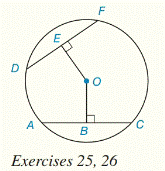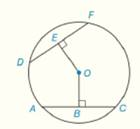Chapter 6.CR, Problem 25CR### Elementary Geometry for College St...

6th Edition
Daniel C. Alexander + 1 other
ISBN: 9781285195698

#### Solutions

Chapter
Section### Elementary Geometry for College St...

6th Edition
Daniel C. Alexander + 1 other
ISBN: 9781285195698
Textbook Problem
1 views

# G i v e n :                               D F - ≅ A C -   i n   ⨀ O                                                                       O E = 5 x + 4                                                                     O B = 2 x + 19 F i n d :                                             O ETo determine

To Find:

The value of OE, if DF-AC- in  O, OE=5x+4 and OB=2x+19Explanation

Approach:

Theorem 6.1.1:

A radius that is perpendicular to a chord bisects the chord.

Theorem 6.1.8:

Congruent chords are located at the same distance from the center of a circle.

Calculation:

Given that DF-AC- in O

So, DF and AC are congruent chords.

So, by Theorem 6.1.8:

Congruent chords are located at the same distance from the center of a circle.

DF and AC are at the same distance from the center of a circle  O.

So, OE=OB

But it is given that, OE=5x+4 and OB=2x+19

So, 5x+4=

### Still sussing out bartleby?

Check out a sample textbook solution.

See a sample solution

#### The Solution to Your Study Problems

Bartleby provides explanations to thousands of textbook problems written by our experts, many with advanced degrees!

Get Started

#### Find more solutions based on key concepts